Editor Ratings:
User Ratings:
[Total: 0 Average: 0]

FinanceSum is a free Windows 8 calculator app which has 264 different financial calculators. The app has categorized these 264 calculators into different categories: Automotive, Budgeting, Credit Card, Housing and Mortgage, Interests, Investments, Loan, Miscellaneous, Small Business, Tax, Wage and Salary. All these categories contain relevant calculators that you will definitely find useful. Some of the calculators are car loan payment calculator, commute cost calculator, auto loan calculator, savings calculator, discount calculator, EMI calculator, Repayment calculator, savings bond calculator, interest calculator, Bond valuation calculator, Mortgage calculator, Currency calculator, vat calculator, and many other types of calculators.

All the calculators have an easy to use interface. You just need to add the relevant values for calculation and your calculation is done quickly.

If you are looking for a particular calculator, then you can even search for it using the built-in search box. It will show the relevant results.## Calculating Finances Using This Windows 8 Calculator App:

You can get FinanceSum app free from Windows store by using the link given at the end of this review.

After launching the app, you will get different categories for calculators:  Automotive, Budgeting, Credit Card, Housing and Mortgage, Interests, Investments, Loan, Miscellaneous, Small Business, Tax, Wage and Salary.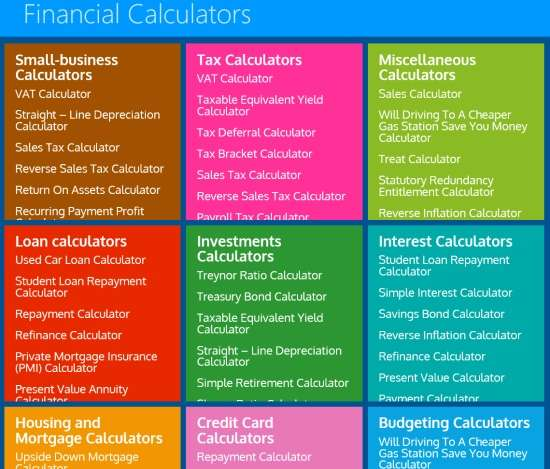Automotive: This category contains calculators for calculating auto/car related finances, like: Auto Loan Calculator, Car Payment Calculator,  Car Finance Calculator, Commute Cost Calculator, etc.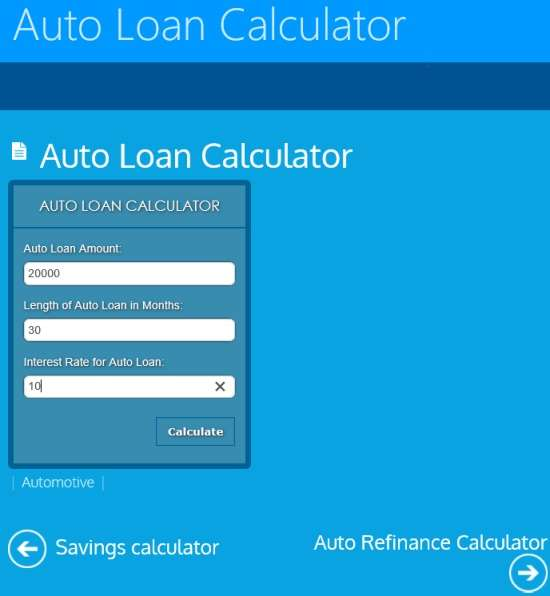Budgeting: This category contains calculators related to budgets, like: Treat calculator, savings calculator, discount calculator, Life insurance calculator, etc.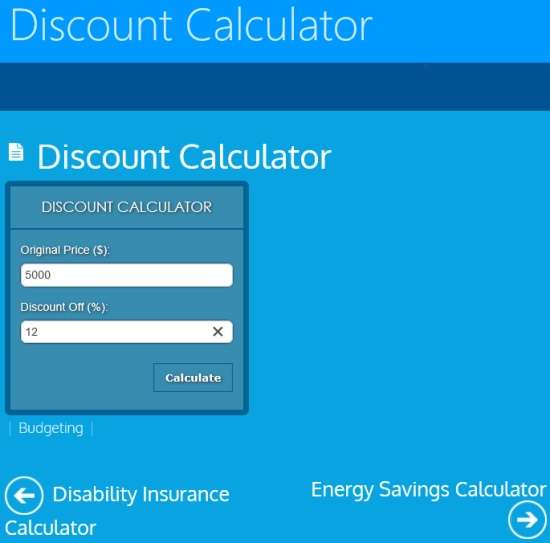Credit Card: As the name suggests, it contains calculators related to credit cards, like: Repayment Calculator, Credit Card Repayment Time Calculator, Credit Card Debt Calculator, etc.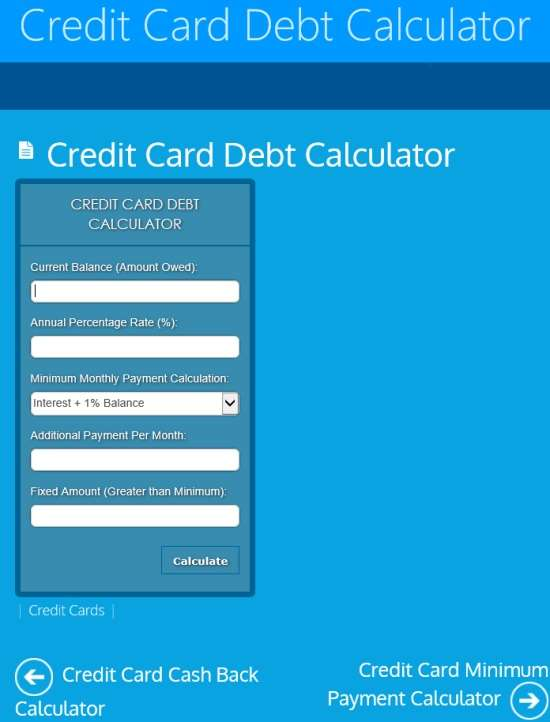Housing And Mortgage: This section contains calculators related to rent and mortgage, like: Total rent calculator, Offer calculator, Mortgage Tax deduction calculator, EMI calculator, Mortgage calculator, 15 year vs 30 year Mortgage comparison calculator, etc.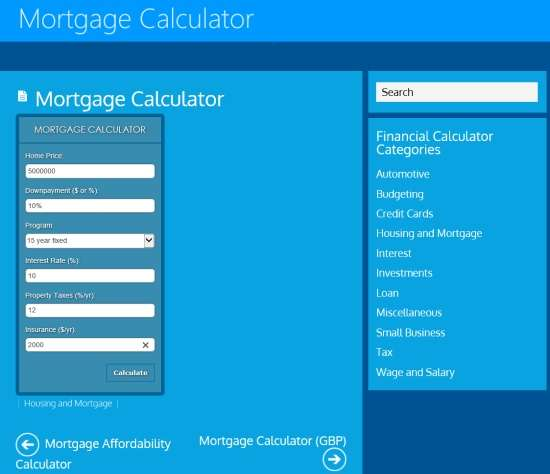Interests: This category contains calculators for calculating different interests, like: Simple Interest Calculator, Inflation Calculator, Interest Calculator, Loan Interest Calculator, Reverse Inflation Calculator, Annual Effective Interest Rate Calculator, etc.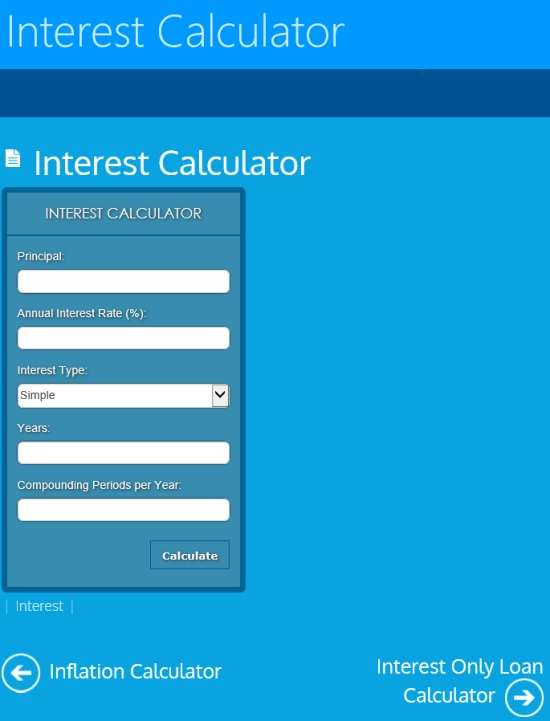Investments: This category contains calculator to calculate various investments, like: Simple Retirement Calculator, Taxable Equivalent Yield Calculator, savings bond calculator, Net Worth Calculator, etc.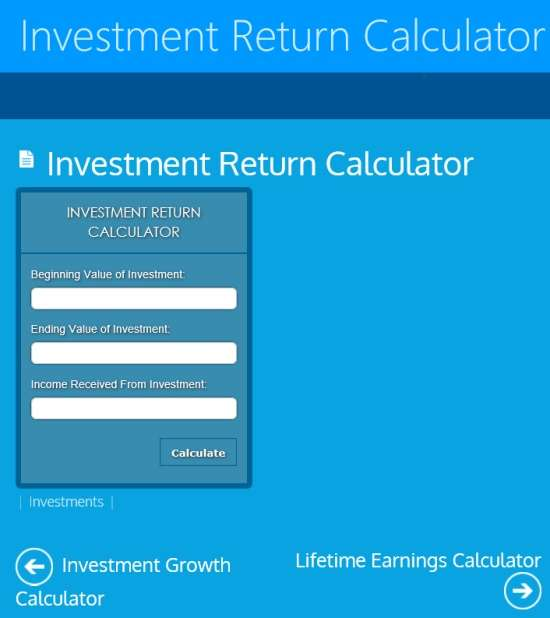Loan: This category contains calculators which can be used to calculate different loans, like: Refinance Calculator, Fixed Loan Calculator, Loan Interest Calculator, Installment Loan Calculator, Payday Loan Calculator, etc.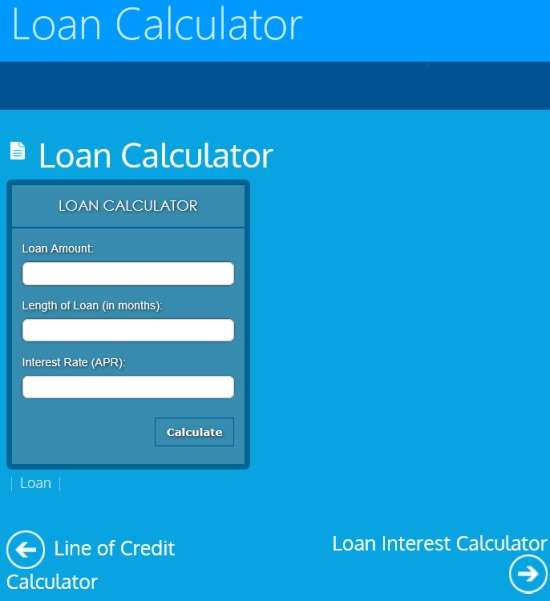Miscellaneous: This category contains many different calculators for different purposes, like: Coinsurance calculator, sales calculator, lifetime earning calculator, etc.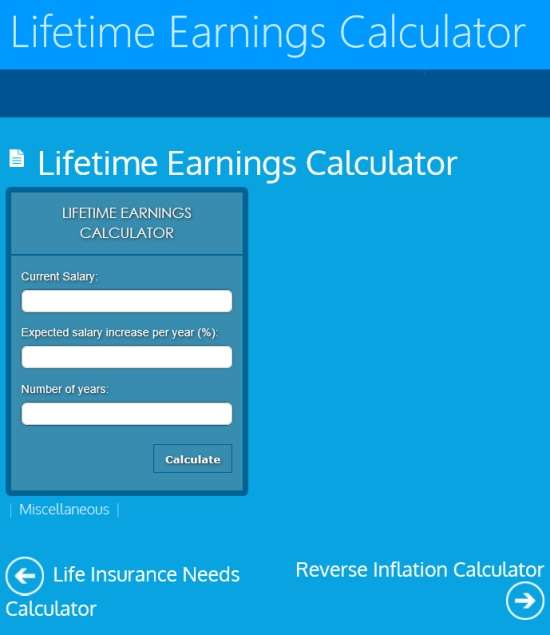Small Business: It contains different calculators that can be used to calculate various business related stuffs, like: VAT calculator, Sales Tax calculator, Profit calculator, Gross Profit Margin Calculator, etc.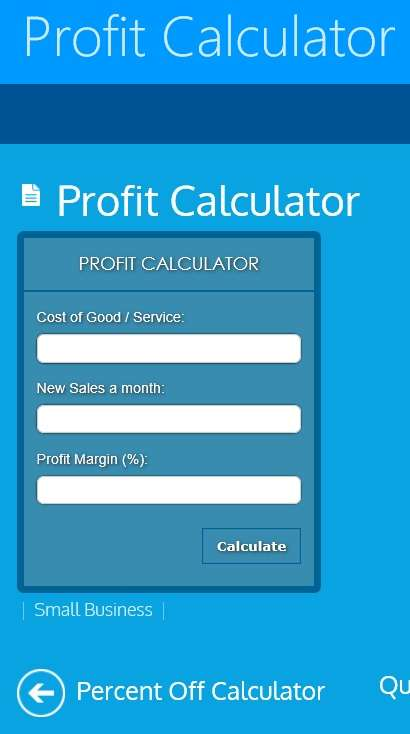Tax: This category contains different calculators that can be used for calculating different taxes, like: Income Tax calculator, payroll tax calculator, sales tax calculator, etc.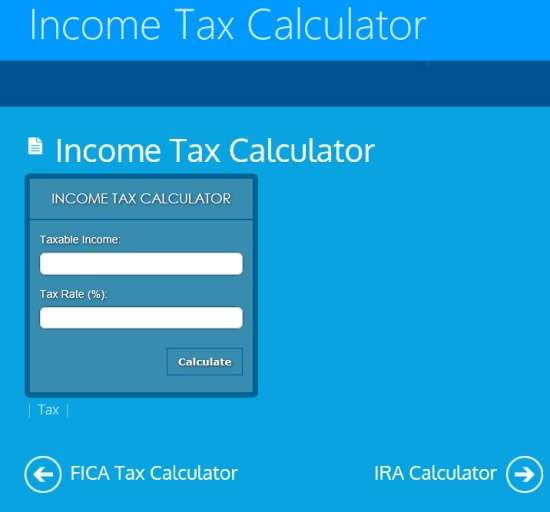Wage and Salary: You can calculate different salary and wages using these calculators, like: Net Pay calculator, Hourly Rate calculator, Income Calculator, Daily Wage calculator, Gross Pay Salary calculator, etc.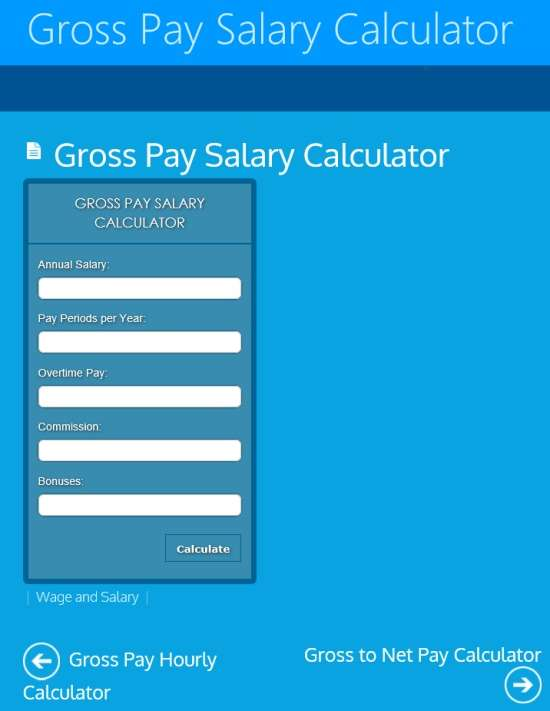Chose any calculator as per your preference, add the values, and click and calculate. It will calculate result in no time. You can even search for any calculator using search box. It will display relevant results.

Also try other calculator apps for Windows 8: 5 free calculators for Windows 8.

## Conclusion:

FinanceSum is an useful finance calculator app for Windows 8. The 264 different calculators for calculating finance for different purposes make this app more useful.